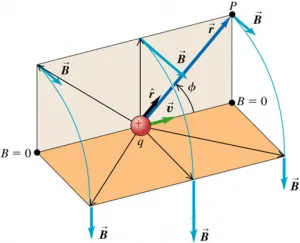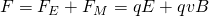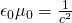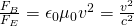# Physics - Electromagnetism - Magnetic field of a moving charged particle## Introduction

Hello it's a me again Drifter Programming! Today we continue with Electromagnetism to get into the first and simplest magnetic field source, which is a moving charged particle. A moving charge of course generates a magnetic field around it, which is what we will be discussing/analyzing today! So, without further do, let's get straight into it!

## Moving charged particle

Until now we saw that charged particles create an electric field around them, which means that other electric charges are affected by their presence. But, this is not the only thing that is generated. Moving charges also create magnetic fields around them. Of course a charge at rest doesn't have a magnetic field, but it still has an electric field. And this is all just a physics rule that we have to accept! There is no way to truly explain this phenomenon. After some more articles we will see that electric and magnetic fields are actually components of something greater, a single entity, which is the so called electromagnetic field. One last thing that we should note is that the charged particle just has to move (needs some velocity), it doesn't have to accelerate (acceleration can be zero)!

Also, don't forget that charged particles are also affected by electric and magnetic field by the so called Lorentz force which is:where the direction of the force (F) can be found using a right hand rule that we covered previously in the series. Of course what's needed now is to see how the magnetic field of a moving charge actually looks like and which mathematical equations it obeys...

## The magnetic field of a moving charged particle

The magnetic field of a single point charge q that is moving with a constant velocity v is given by the equation:vector and "magnitude" form.

The component μ0 is of course the permeability of free space and is connected with the permittivity of free space (ε0) by the equation:where c is the speed of light!

The magnetic field is perpendicular to the velocity of the particle and so to the plane that contains the magnetic field source point (cross product between vectors). For a point charge moving with velocity v, the magnetic field lines are circles centered on the line of vector v and lying in planes perpendicular to this line. To find the direction in which these "circular" magnetic field lines go we use another right hand rule. For positive charges the thumb shows the velocity (or trajectory) of the charged particle and the "curling" of the other fingers shows the direction of the field lines (clockwise or not). For negative charges the direction of the lines is opposite or we could just use our left hand :P

## Parallel charged particles

Let's now consider two charged particles. Till now we saw that they interact (forces) because of the electric fields, which makes them attract or repel. When those charges are not moving then they don't have an magnetic field, but as soon as they move because of the electric forces they of course also start having magnetic fields. Let's consider that they have the same velocity and charge, and that they move in parallel trajectories. The forces applied to each are:where v0 is the initial velocity of the particles (it changes after the interaction).

Supposing that the particles are both protons (positive) the two forces add up "outwards", cause they repel each other. That way the total force applied on each particle, because of the interaction with the other particle is:But, what is more interesting is the ratio of the forces which is:where c is of course the speed of light and so the only thing needed is the velocity of the particle. So, the electric or magnetic force can be found, when knowing the other. Something very useful, cause that way electric and magnetic fields are now "kinda" connected. A step closer to electromagnetic fields!

## REFERENCES:

Mathematical equations that I had in this post where drawn using quicklatex!

### Electric fields:

Getting into Electromagnetism -> electromagnetim, electric charge, conductors, insulators, quantization

Coulomb's law with examples -> Coulomb's law, superposition principle, Coulomb constant, how to solve problems, examples

Electric fields and field lines -> Electric fields, Solving problems around Electric fields and field lines

Electric dipoles -> Electric dipole, torque, potential and field

Electric charge and field Exercises -> examples in electric charges and fields

### Electric flux:

Electric flux and Gauss's law -> Electric flux, Gauss's law

Applications of Gauss's law (part 1) -> applying Gauss's law, Gauss applications

Applications of Gauss's law (part 2) -> more Gauss applications

Electric flux exercises -> examples in electric flux and Gauss's law

### Electric potential:

Electric potential energy -> explanation of work-energy, electric potential energy

Calculating electric potentials -> more stuff about potential energy, potential, calculating potentials

Millikan's Oil Drop Experiment -> Millikan's experiment, electronvolt

Cathode ray tubes explained using electric potential -> cathode ray tube explanation

Electric potential exercises (part 1) -> applications of potential

Electric potential exercises (part 2) -> applications of potential gradient, advanced examples

### Capacitance:

Capacitors (Condensers) and Capacitance -> Capacitors, capacitance, calculating capacitance

How to solve problems around Capacitors -> combination, solving problems, simple example

Electric field energy and density -> Electric field energy, energy density

Dielectric materials -> Dielectrics, dielectric constant, permittivity and strength, how to solve problems

Electric capacitance exercises -> examples in capacitance, energy density and dielectrics

### Current, resistance and EMF:

Electric current -> Electric current, current density

Electrical resistivity and conductivity -> Electrical resistivity, conductivity, thermal coefficient of resistivity, hyperconductivity

Electric resistance -> Resistance, temperature, resistors

Electromotive Force (EMF) and Internal resistance -> Electromotive force, internal resistance

Power and Wattage of Electronic Circuits -> Power in general, power/wattage of electronic circuits

Electric current, resistance and emf exercises -> exampes in all those topics

### Direct current (DC) circuits:

Resistor Combinations -> Resistor combinations, how to solve problems

Kirchhoff's laws with applications -> Kirchhoff's laws, how to solve problems, applications

Electrical measuring instruments -> what are they?, types list, getting into some of them, an application

Electronic circuits with resistors and capacitors (R-C) -> R-C Circuit, charging, time constant, discharging, how to apply

RC circuit exercises -> examples in Kirchhoff, charging, discharging capacitor with/without internal resistance

### Magnetic field and forces:

Magnetic fields -> Magnetism, Magnetic field

Magnetic field lines and Gauss's law of Magnetism -> magnetic field lines, mono- and dipoles, Flux, Gauss's law of magnetism

The motion of charged particles inside of a magnetic field -> straight-line, spiral and helical particle motion

Applications of charged particle motion -> CERN, Cyclotrons, Synchrotrons, Cavity Magetron, Mass Spectrometry and Magnetic lens

Magnetic force applied on Current-Carrying Conductors -> magnetic force on current-carrying conductors/wires, proofs

Magnetic force and torque applied on current loops (circuits) -> magnetic force on current loops, magnetic moment and torque

Explaining the Physics behind Electromotors -> tesla, history and explaining the physics behind them

Magnetic field exercises -> examples in magnetic force, magnetic flux, particle motion and forces/torque on current-carrying conductors

And this is actually it for today's post! Next time we will get into the magnetic field created by straight-line current-carrying conductors, which of cousre is another source of magnetic fields.

C ya!

H2
H3
H4
3 columns
2 columns
1 column